## Probability: Proportions

The proportion of items in a population that have some specified characteristic is the quotient of the number of items in the population with that characteristic divided by the population size. We use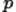to represent the population proportion.

The proportion of items in a sample that have some specified characteristic is the quotient of the number of items in the sample with that characteristic, x, divided by the sample size. We use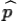, read as "p hat", to represent the sample proportion. In symbols we have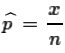As we might expect, if we took repeated samples of size n then the mean of the sample proportions would approach the population proportion. That is,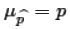It would also be the case that the standard deviation of the sample proportions would approach the following value: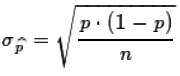There is an interplay between the size of p and the size of n such that in special cases, hopefully in any case we use, if n*p ≥ 10 and n*(1-p) ≥ 10 then the distribution ofis approximately normal with mean=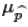and standard deviation=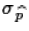. That approximation means that, in those cases, we can use the normal table and/or the R functions pnorm() and qnorm() to answer questions about the probability of certain random events involving proportions in random samples of a population.

For example, if we know that 74% of the students in introductory engineering courses are males, then, in a random sample of 94 students in such classes what is the probability that the sample will have 80% or more males? The 74% in the population makes p=0.74. That makes (1-p)=0.26. With n=94 we confirm that np≥10 and n(1-p)≥10, with values of 69.56 and 24.44, respectively. Therefore, we can use a normal approximation with μ=p=0.74 and σ=sqrt(p(1-p)/n)=sqrt(0.74*0.26/94)≈0.04524. In order to use the standard normal table we need to change the 80% to a z-score,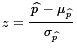, to get z=(0.80-0.74)/0.04524≈1.326. Then, look at the standard normal table, a portion of which is shown in Figure 1. We cannot find our z value, 1.326, exactly in the table because the table is only good for 3 places. The best the table can do is to get us close to 1.326. That is, we can find values for 1.32 and 1.33. From the table we see that the area to the left of 1.32 is about 0.9066 while the area to the left of 1.33 is about 0.9082.

Figure 1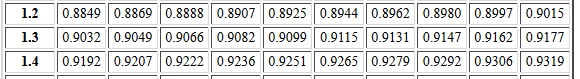For 1.326 we want to move 6 tenths of the way from the former toward the latter. 0.9082 - 0.9066 = 0.0016, and 0.6*0.0016=0.00096 so our answer for the area to the left of 80% would be 0.9066+0.00096=0.90756. Of course, we want the area to the right of that value and that would be 1 - 0.90756 = 0.09244. Therefore, the probability of having 80% or more males is about 9.244%, remembering that we had to use various rounded values.

Then again, because we have been paying attention and because we have R, we could just form the command pnorm(0.80, mean=0.74, sd=sqrt(0.74*0.26/94), lower.tail=FALSE), as shown in Figure 2, and get the same, though a bit more accurate, result. In either case we are not going to report all the extra digits. We would round both answers to say that the probability is 9.24%.

Figure 2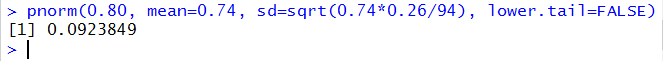Clearly, using the pnorm() function in R is much easier than going through all of that work getting the z-score, looking up values, interpolating, and then, in this case, getting the complementary value. Still, we would have to remember just how to form that pnorm() command. Then again, the form does not change, just the values that we are using. Those values are the sample proportion, the population proportion, the sample size, and do we want to use the left or the right tail? Why not just create our own function for this. We could do something like
```pprop <- function( phat, p, n, lower.tail=TRUE)
{
if(p*n < 10) {return("n*p < 10, will not compute this")}
if(n*(1-p) < 10)
{return("n*(1-p)<10, will not compute this")}
psd <- sqrt( p*(1-p)/n)
prob <- pnorm(phat, p, psd, lower.tail)
return( prob )
}
```
And then the problem becomes one of just giving the command pprop(.80, .74, 94, lower.tail=FALSE). All of this is shown in Figure 3.

Figure 3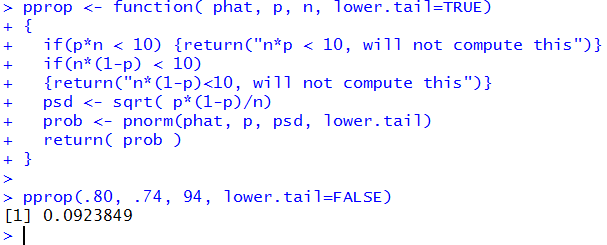You might note that the function definition uses lower.tail=TRUE. This was done to keep pprop() similar to pnorm() and pt(). Also, our function saves us from trying to apply the approximation in cases where it does not fit.

Were we to have a case where 74% of the students were male and we had a sample of size 36, then the value of 36*(1-0.74) would not be greater than or equal to 10. That tells us that we cannot apply the normal approximation, no matter what sample proportion we want to evaluate. To find the probability that the sample has 60% of fewer males we would give the command pprop(.60, .74, 36) which would just return the message "n*(1-p)<10, will not compute this" as shown in Figure 4.

Figure 4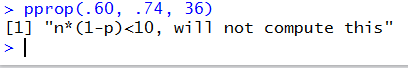Some note should be made here about problem statements that do not give you the probabilities for having the characteristic or not having it, that is, they do not give you p and therefore (1-p). For example, we might be told that from a population of 1256 individuals we have a sample that contains 13 males and 21 females. Could we use or normal approximation for proportions in this case? First, we note that our sample size is 34 (the total of males and females). 34 is less than 5% of our population (0.05*1256 is 62.8). We need to be sampling less than 5% in order to ignore the changes in probabilities when we sample without replacement. Second, if we knew the proportion of males, call it pm, and the proportion of females, call it pf, so pm+pf=1, then we would need n*pm≥10 and n*pf≥10 in order to use the normal approximation. Since we do not know pm or pf we will use 15/34 for pm and 21/34 for pf. Then our requirement that n*pm≥10 becomes 34*(15/34)≥10 and our requirement that n*pf≥10 becomes 34*(21/34)≥10. Note that in both cases the 34's cancel and what is left is that the number of males must be greater than or equal to 10 and the number of females must be greater than or equal to 10. In short, looking at the original problem statement we did not have to go through all that computation, we just needed to be sure that we had at least 10 items with the characteristic and at least 10 items without the characteristic.

Here are three more examples, but we will use pprop() to solve them.

Figure 5 gives data taken from the Center for Disease Control web page.

Figure 5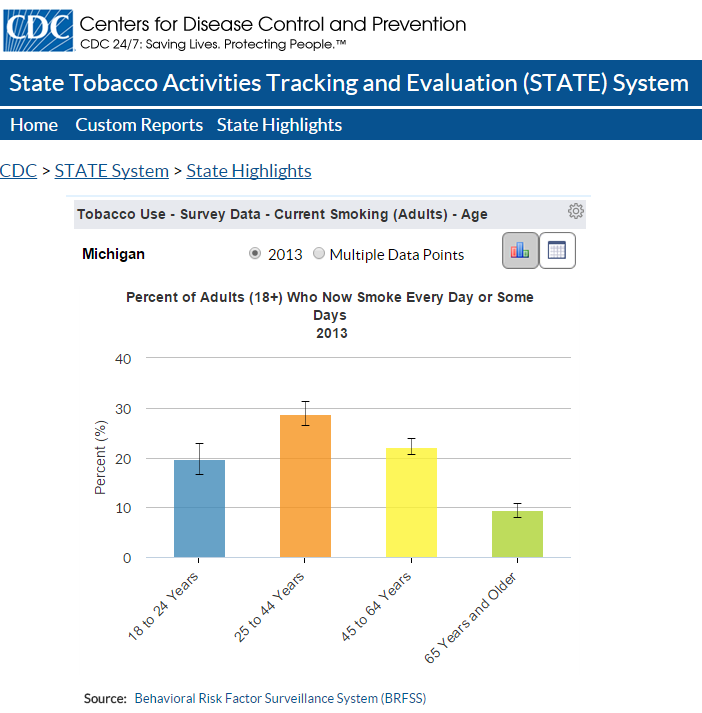According to Figure 5 about 20% of people ages 18 to 24 years in Michigan reported smoking every day or some days in 2013. In a random sample of size 36 of people who lived in Michigan in 2013 and who were 18 to 24 then, what is the probability that 5 or fewer of them smoked every day of some days? We will just fill in the pprop() statement to do this. We start by typing, in our R session, pprop(. R immediately fills in the closing parenthesis and shows a helpful guide giving the function parameters. This is shown in Figure 6

Figure 6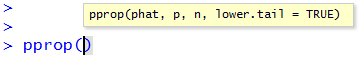We see that the first parameter is phat. To supply this we need to type 5/36. The second and third parameters are p and n, for which we supply .20 and 36. The fourth parameter shows that its default value is TRUE. This is the value that we want so we do not even need to supply it. Thus our complete command becomes the pprop(5/36, 0.20, 36) shown, with the result, in Figure 7.

Figure 7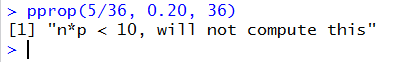The system has saved us from making a mistake!

If we change the problem, slightly, and ask in a sample of size 72 of these same people what is the probability of getting 10 or fewer smokers, then the command becomes the pprop(10/72, 0.20, 72) shown, with the result, in Figure 8.

Figure 8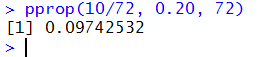It is interesting to note that we doubled the sample size and left the proportion the same (5/36=10/72) but now we have enough in the sample to use the approximation.

Figure 9 presents data taken from another CDC page.

Figure 9If 31% of all traffic-related deaths in 2013 were in alcohol-impaired driving crashes and we take a sample of 58 driving crashes from 2013, what is the probability that between 15 and 45 of those crashes will have been deemed alcohol-impaired? We will do this by saying that the answer will be P(X ≤ 45) - P(X < 15).

Wait a minute! Why use ≤ in one place and < in the other? This immediately opens our eyes to an issue that we ignored in doing the previous problem. In particular, since the normal distribution is continuous, we recall that the P(X = 15)=0. Our first analysis seems flawed. We should restate the problem as P(X < 45.5) - P(X < 14.5). We should have done a similar change in the previous problem, but we are just too lazy to go back and fix that. Well maybe we can sneak an update into the answer to this one, shown in Figure 10.

Figure 10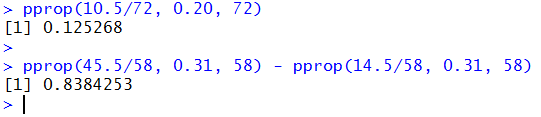Figure 10 gives an improved interpretation to the earlier problem (resulting in changing that answer to 12.5%), and an answer to this problem, namely, 83.84%.

The information in Figure 11 was taken from the Bureau of Transportation Statistics.

Figure 11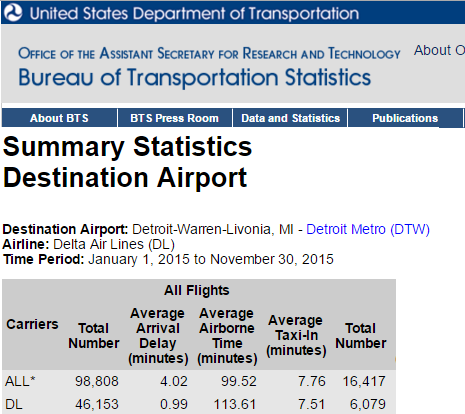With that information in mind, for a sample of 85 random Delta flights between 01/01/2015 and 11/30/2015 with a destination of Detroit (DTW), what is the probability that there will be less than 5 or more than 80 delayed flights in the sample? We use the pprop() to do this as the sum of the probability of the two cases, taking into account the offset between whole number values. This is shown in Figure 12.

Figure 12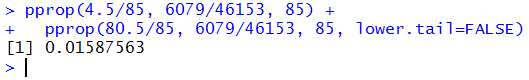Those are pretty extreme values, so we are not shocked to see such a small probability, 1.588%, as the answer.